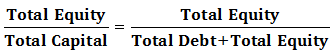# Proprietary Ratio

Proprietary Ratio is a type of Solvency Ratio that determines the relative contribution of Proprietor/Shareholder in a company’s Total Capital. Shareholders Contribution is termed as Equity. The proprietary Ratio is also known as the Net Worth Ratio or Equity Ratio.
$$Proprietary\quad Ratio=\frac { Total\quad Equity }{ Total\quad Capital }$$ Total Equity = Total Assets - Total Liability;
total assets and total liability include current as well as non-current assets and liabilities, respectively. It also includes Reserves and Surplus of the company.Where,
• And Total Capital = Total Equity + Total Debt; total capital implies shareholders as well as creditors contribution.

## Significance and Interpretation

• Proprietary Ratio =• For, Proprietary Ratio = 1, Total Debt must be Zero, and Debt cannot be less than zero.
• Hence, the Maximum Value of Proprietary Ratio = 1
• Proprietary Ratio = 0.5: This implies that the Total Equity of a company is half of the total assets owned or in other words Total Debt = Total Equity.
• Proprietary Ratio < 0.5: This implies that Equities contribute less than 50% in the company’s total assets or in other words Total Debt exceeds total equity of a company, the company faces lots of issues in times when the interest rates rise.
• Proprietary Ratio > 0.5: This implies that equities dominate in the company’s total capital and they constitute more than 50% of the total capital.
• The ideal Proprietary Ratio > 0.5, which indicates that the company has more than half of the capital as equity. However, it must be noted that this limit may shift depending upon the regulatory reforms and/or type of business.
• A high Proprietary Ratio is beneficial for lenders to the company, wherein a low Proprietary Ratio is beneficial to the company for trading in Equities.

### Examples

#### Example 1:

M/S ABC Ltd. reported total debts worth ₹380 Crores and total equity as ₹620 Crores, find the proprietary ratio of M/S ABC Ltd

#### Solution:

Total Equity = ₹620 Crore; Total Debt = ₹380 Crore
Total Capital = Total Debt + Total Equity
(380 + 620)
₹1000 Crore
Hence, Proprietary Ratio = Total Equity / Total Capital
620/1000
31/50 or 0.62

#### Example 2:

The following information is available about M/S XYZ Ltd, find a proprietary ratio of the firm.
Sr. No Particulars Amount (in ₹ Cr)
1 Current Liability 440.00
2 Non-Current liability 50.00
3 Share Capital 150.00
4 Money Reserved Against Share Warrants 80.00
5 Reserves and Surplus 500.00

#### Solution:

Total Equity = Share Capital + Money Reserved Against Share Warrants + Reserves and Surplus
₹730 Crore
Total Debt = Current Liability + Non- Current Liability
₹490Crore
Total Capital = Total Debt + Total Equity
₹1220 Crore
Proprietary Ratio = Total Equity/ Total Capital
730/1220
73/122
Hence, Proprietary Ratio = 73/122 or 0.56